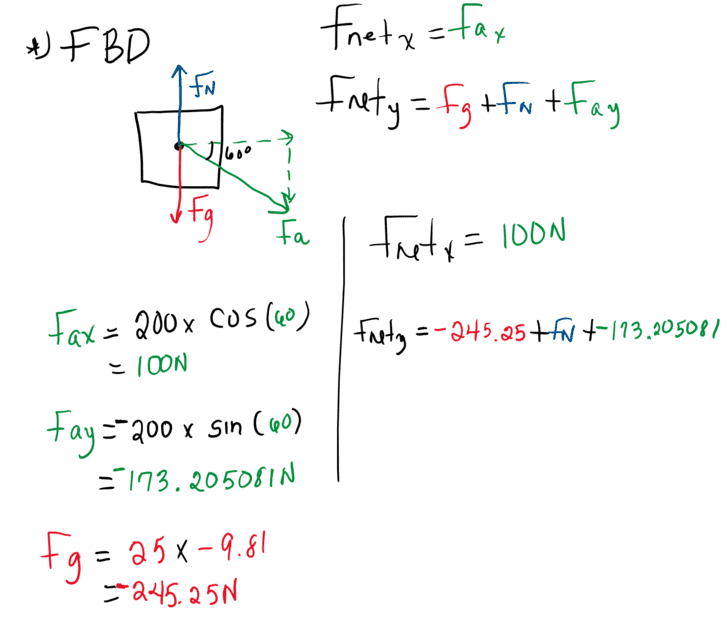# Acceleration & Normal Force of a Box: 4 m/s2 & 418 N

• Remle

#### Remle

Homework Statement
A 25 kg box is being pushed down on by an applied force of 200 N inclined at 60° below the horizon.
Relevant Equations
Normal force: FN=m×g
1. What is the acceleration of the box? Paper says the answer is 4 m/s2.
2. What is the Normal force acting on the box? Paper says the answer is 418 N.
I know that for most cases FN=Fg=W. So, by definition the "original" Normal force is 245.25 N (am I correct?)
I calculated the Fay which is -173.205081 N, so I think that I have to add this two? Then the "new" FN and Fg would be 418 N.

Also when I do my calcs for acceleration I end up with 8 m/s2 not 4.

This is what I have:This box must be on some kind of surface to have a normal force exerted on it. Is the surface horizontal or inclined. What does the problem say? Your answers will depend on that clarification.

What you call the "original" normal force is the weight. So call it that to avoid confusion.
I calculated the Fay which is -173.205081 N, so I think that I have to add this two?
Which two? Also, please limit your numbers no more than 3 significant figures.

•Lnewqban
This box must be on some kind of surface to have a normal force exerted on it. Is the surface horizontal or inclined. What does the problem say? Your answers will depend on that clarification.
The problem does not say anything about a surface, so I just assumed it because that's how a normal force works.
What you call the "original" normal force is the weight. So call it that to avoid confusion.
When I say original is I calculate FN with mass and gravity. Then I start adding or substracting other forces (if there's any) to this FN.
Which two? Also, please limit your numbers no more than 3 significant figures.
Fg=245.25N and Fay=-173.205 N

I end up with 8 m/s2 not 4.
How ?

How ?
$$F_\rm{a_\rm{y}} = -200 \times \sin(60) = -173.205~N$$
$$F_\rm{a_\rm{x}} = 200 \times \cos(60) = 100~N$$
$$F_\rm{g}= 25 \times -9.81=-245.25~N$$
$$F_\rm{Net_\rm{y}} = - 245.25 - 173.205 + 245.25 = -173.205~N$$
$$F_\rm{Net_\rm{x}} = 100~N$$
$$F_\rm{Net}=\sqrt{{(-173.205)^2} + 100^2} = 200.00~N$$
$$a = \frac{200} {25} = 8~\rm{m/s^2}$$

$$F_\rm{Net_\rm{y}} = - 245.25 - 173.205 + 245.25 = -173.205~N$$
The normal force doesn't automatically equal the weight of the box. It is a force acting on the box, which you must solve for.

•Chestermiller
$$F_\rm{Net}=\sqrt{{(-173.205)^2} + 100^2} = 200.00~N$$

You are reconstructing the given 200 N! Do you think the acceleration is in that direction ?

 PS: kudos for the typesetting !

##\ ##

I know that for most cases FN=Fg=W
Confuses you. Not useful.

The ##F_\text{net}## concept also confuses you. Suggestion: memorize Newton in the form $$\vec F_\text{net} = m\vec a$$

In your exercise there is a box on a smooth horizontal surface. Without the 200 N extra force the net force is zero: the weight force from gravity is balanced by the normal force. So ##\vec a = 0##.

With the 200 N extra force , you correctly look at its components. And write ##F_\text{net, y}= F_{\text g} + F_\text{a, y} + F_\text{N}\ .\ ## Since there is no acceleration in the vertical direction, we must have ##F_\text{net, y}=0##. Which allows you to calculate the normal force.

In the horizontal direction, you (also correctly) write ##F_\text{net, x}= F_\text{a, x} \ . \ ## There are no other horizontal forces (no friction), so ##m\,a_x = F_\text{a, x} \ .\ ## Which allows you to calculate the acceleration.

That's all there is to it!

##\ ##

•hutchphd, Lnewqban and Remle
Confuses you. Not useful.

The ##F_\text{net}## concept also confuses you. Suggestion: memorize Newton in the form $$\vec F_\text{net} = m\vec a$$

In your exercise there is a box on a smooth horizontal surface. Without the 200 N extra force the net force is zero: the weight force from gravity is balanced by the normal force. So ##\vec a = 0##.

With the 200 N extra force , you correctly look at its components. And write ##F_\text{net, y}= F_{\text g} + F_\text{a, y} + F_\text{N}\ .\ ## Since there is no acceleration in the vertical direction, we must have ##F_\text{net, y}=0##. Which allows you to calculate the normal force.

In the horizontal direction, you (also correctly) write ##F_\text{net, x}= F_\text{a, x} \ . \ ## There are no other horizontal forces (no friction), so ##m\,a_x = F_\text{a, x} \ .\ ## Which allows you to calculate the acceleration.

That's all there is to it!

##\ ##
You are reconstructing the given 200 N!
OMG... feel dumb. The ## 200~N ## is already the diagonal force. So the only thing I have to do is find ## F_\rm{ay} ## and it add to ## F_\rm{g} ##. Then only force left is ## F_\rm{ax} ## which that gives me ## 100~N ## dividing that with ## 25~\rm{kg} ## provides the ## 4~\rm{m/s^2} ##.

Thank you BvU. Just need another mind to help me see things differently.

•berkeman, BvU and Lnewqban
 PS: kudos for the typesetting !

##\ ##
@Remle ,And I also say "kudos for the typesetting!"

•Remle and erobz